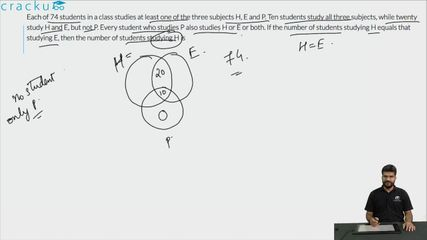Question 84

# Each of 74 students in a class studies at least one of the three subjects H, E and P. Ten students study all three subjects, while twenty study H and E, but not P. Every student who studies P also studies H or E or both. If the number of students studying H equals that studying E, then the number of students studying H is

Solution

Let us draw a Venn diagram using the information present in the question.

It is given that the number of students studying H equals that studying E.

Let 'x' be the total number of students who studied H, and H and P but mot E.We can also say that the same will be the number of students who studied E, and E and P but not H.Therefore,

x + 20 + 10 + x = 74

x = 22

Hence, the number of students studying H = 22 + 10+ 20 = 52

### View Video Solution• All Quant CAT Formulas and shortcuts PDF
• 30+ CAT previous papers with solutions PDF